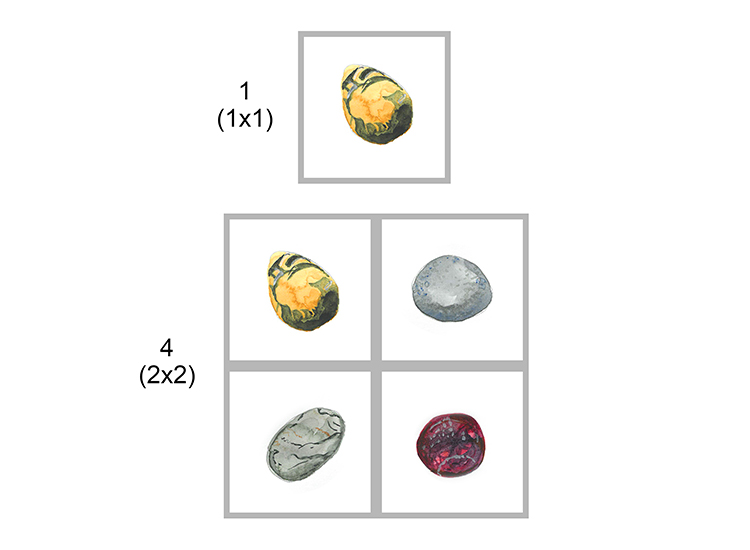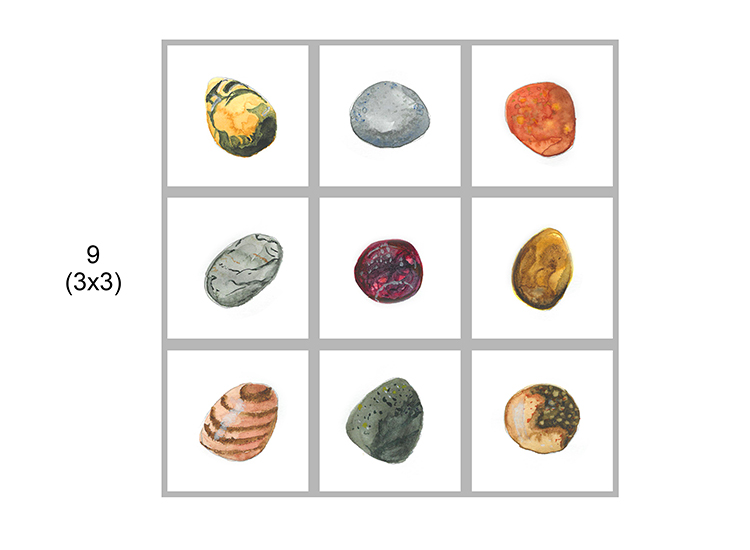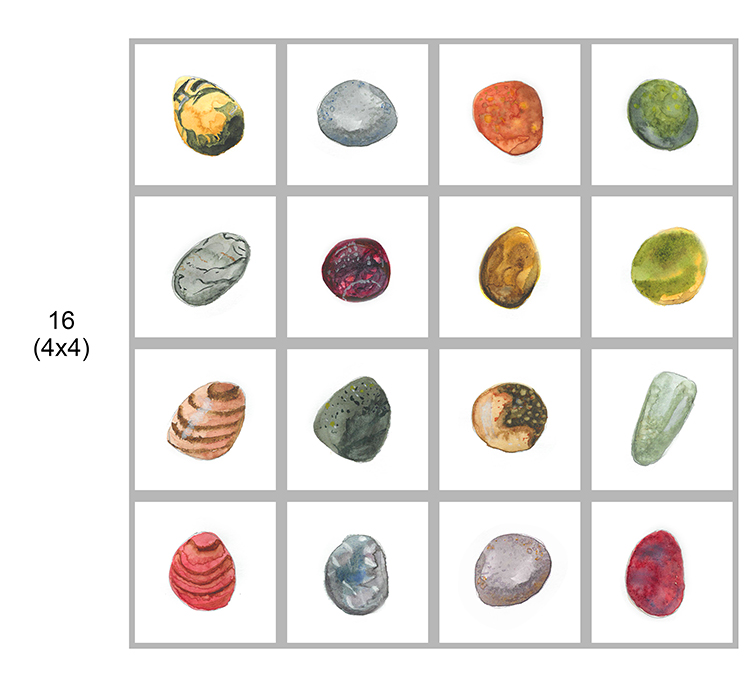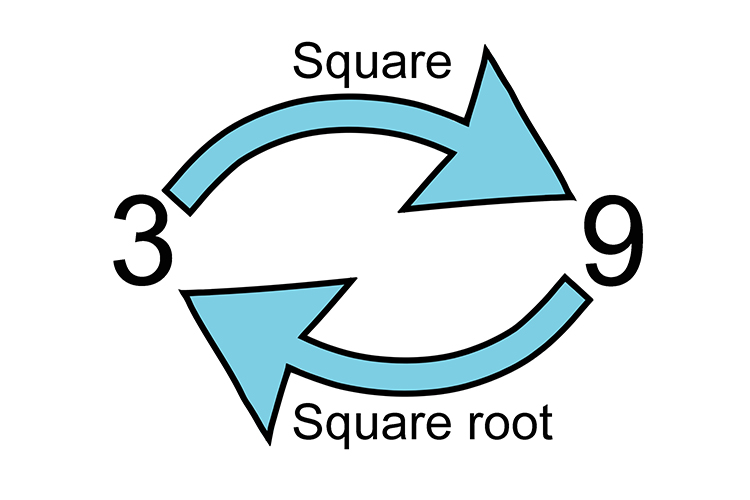# Squared numbers

## Square number = Any number squared is multiplied by itself (22 = 2 x 2)Square numbers are…..square, see below (using pebbles).See how each square grows. We extend each side and then fill out all the remainder.

Here are the first 10 numbers squared:

 1^2 = 1times1 = 1 2^2 = 2times2 = 4 3^2 = 3times3 = 9 4^2 = 4times4 = 16 5^2 = 5times5 = 25 6^2 = 6times6 = 36 7^2 = 7times7 = 49 8^2 = 8times8 = 64 9^2 = 9times9 = 81 10^2 = 10times10 = 100

Example 1

5^2=5times5=25

Example 2

(-5^2)=-5times-5=25

(When we square a negative number we get a positive result)

Example 33 squared is 9 just as the square root of 9 is 3

Example 4

(3/4)^2=3/4times3/4=(3times3)/(4times4)=9/16